# RD Sharma Solutions for Class 6 Maths Chapter 9: Ratio, Proportion and Unitary Method Exercise 9.1

The ratio of two quantities of the same kind and in the same units is a fraction that shows how many times the one quantity is of the other. This exercise has questions based on ratio and their comparison by difference and division. RD Sharma Solutions Class 6 help students understand the steps which are followed in converting the given ratio to its simplest form. The students can download RD Sharma Solutions for Class 6 Maths Chapter 9 Ratio, Proportion and Unitary Method Exercise 9.1 PDF which are given here.

## RD Sharma Solutions for Class 6 Maths Chapter 9: Ratio, Proportion and Unitary Method Exercise 9.1 Download PDF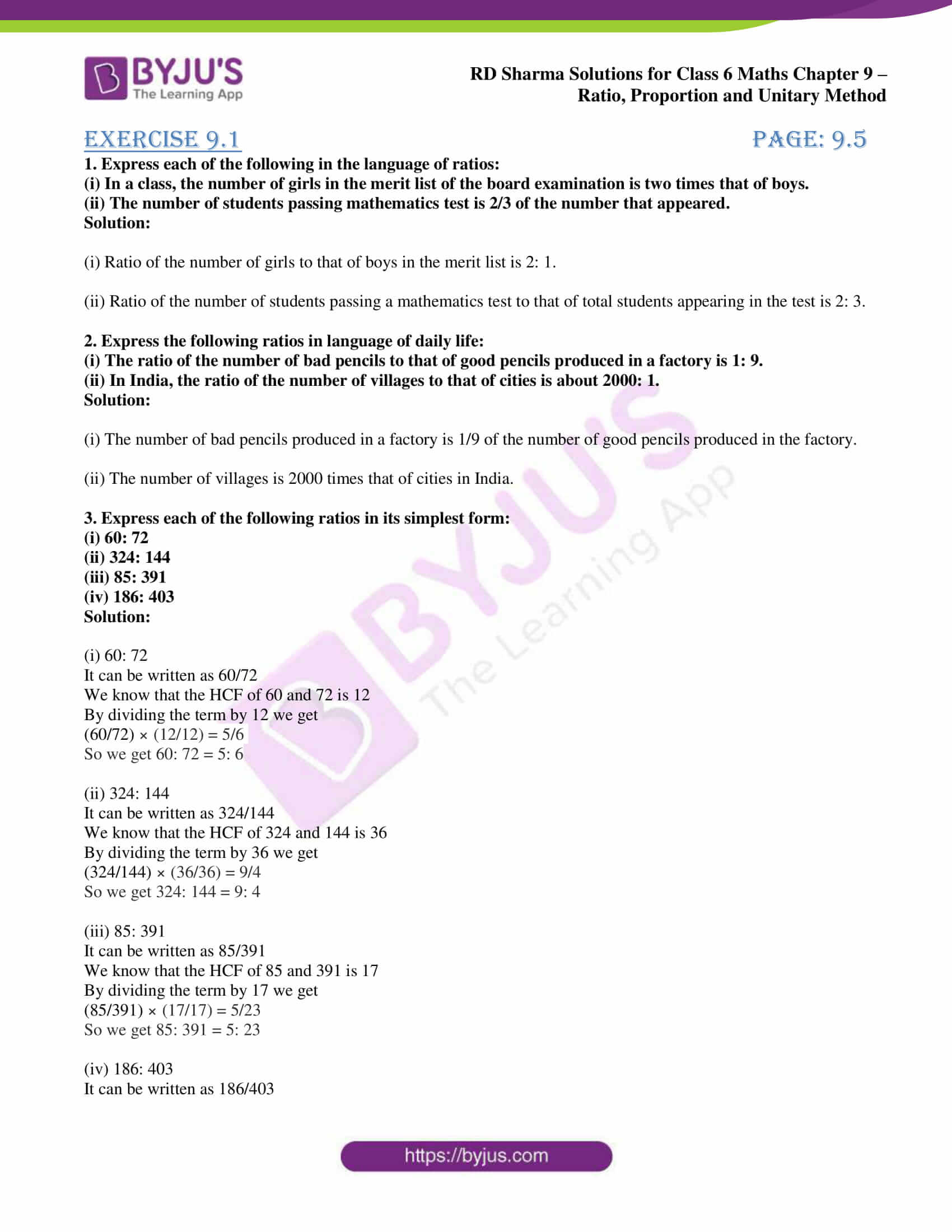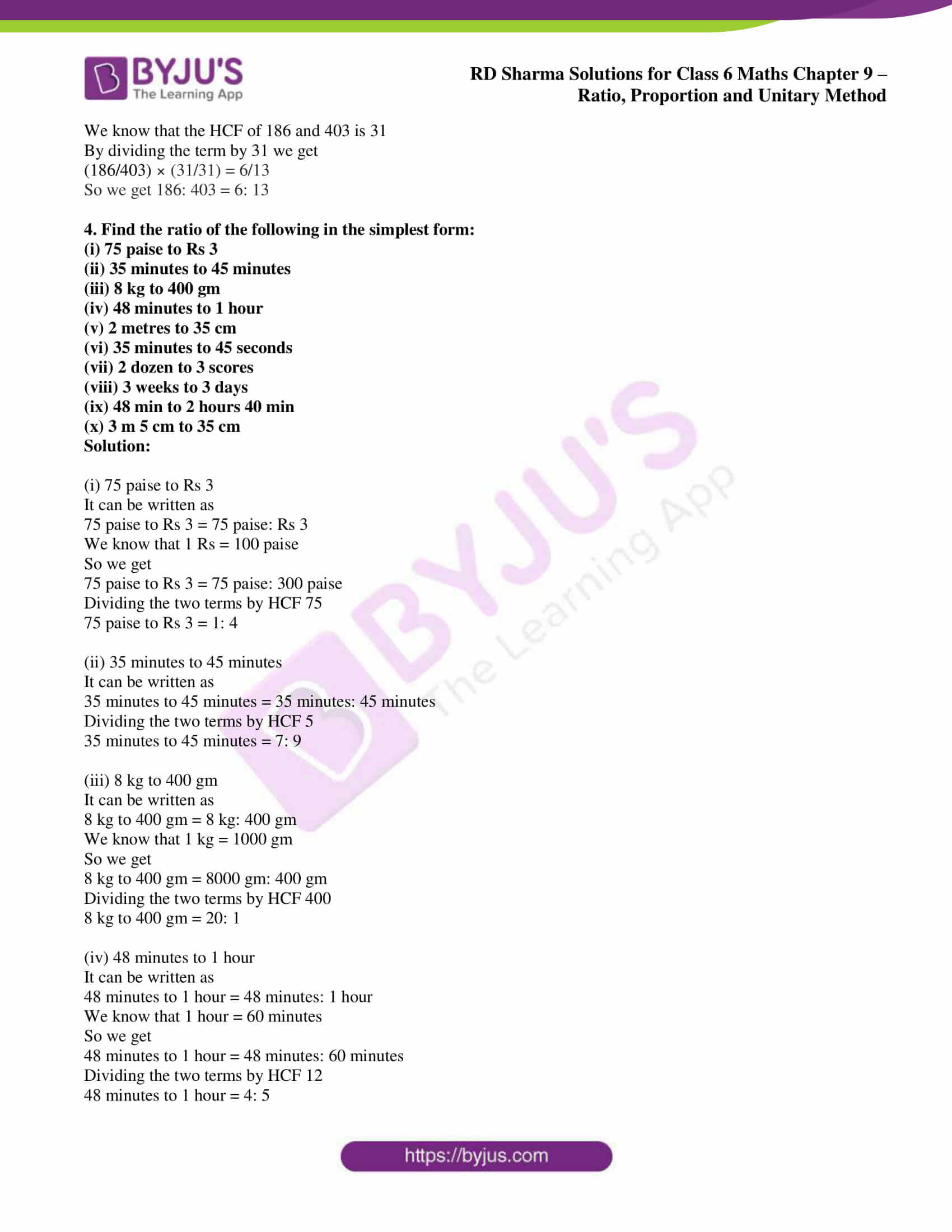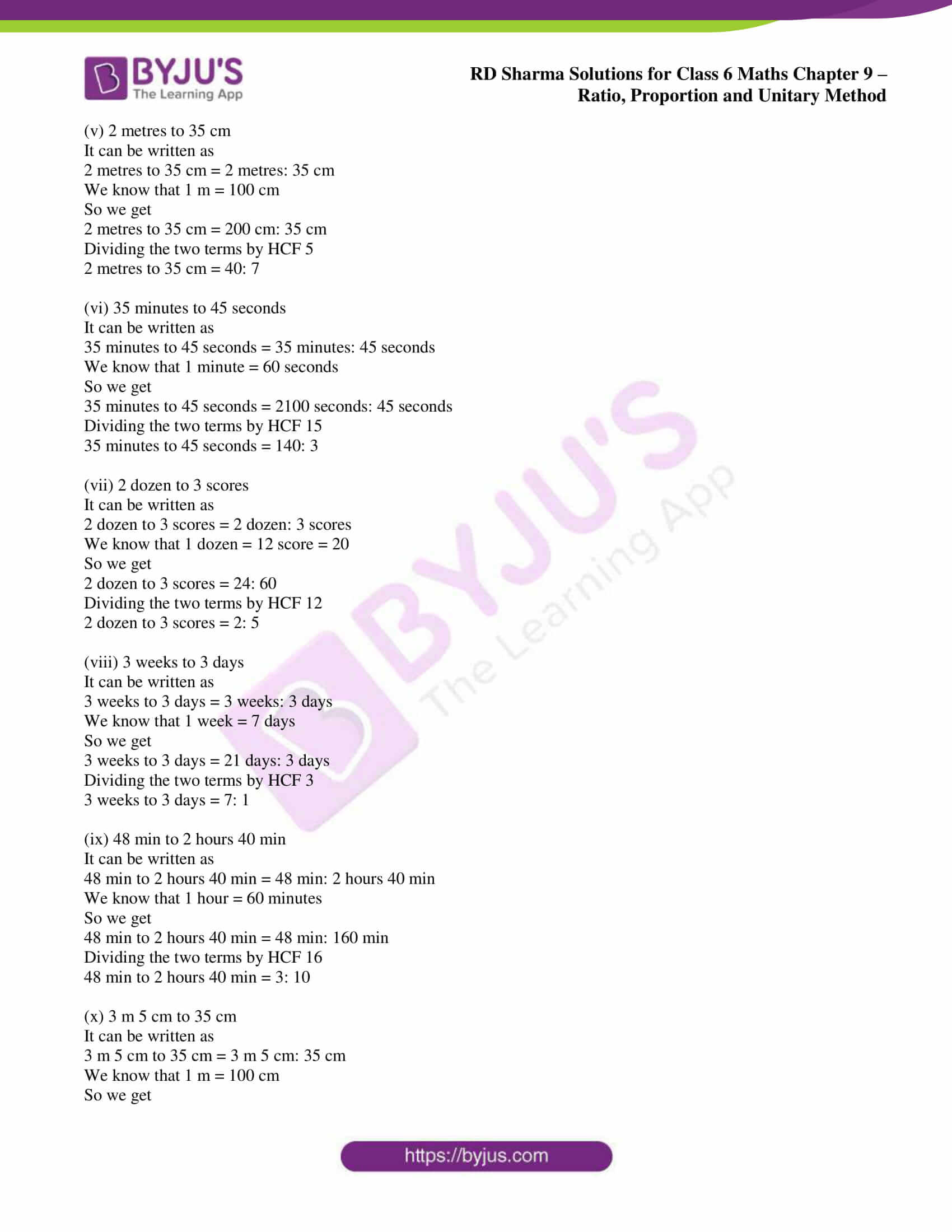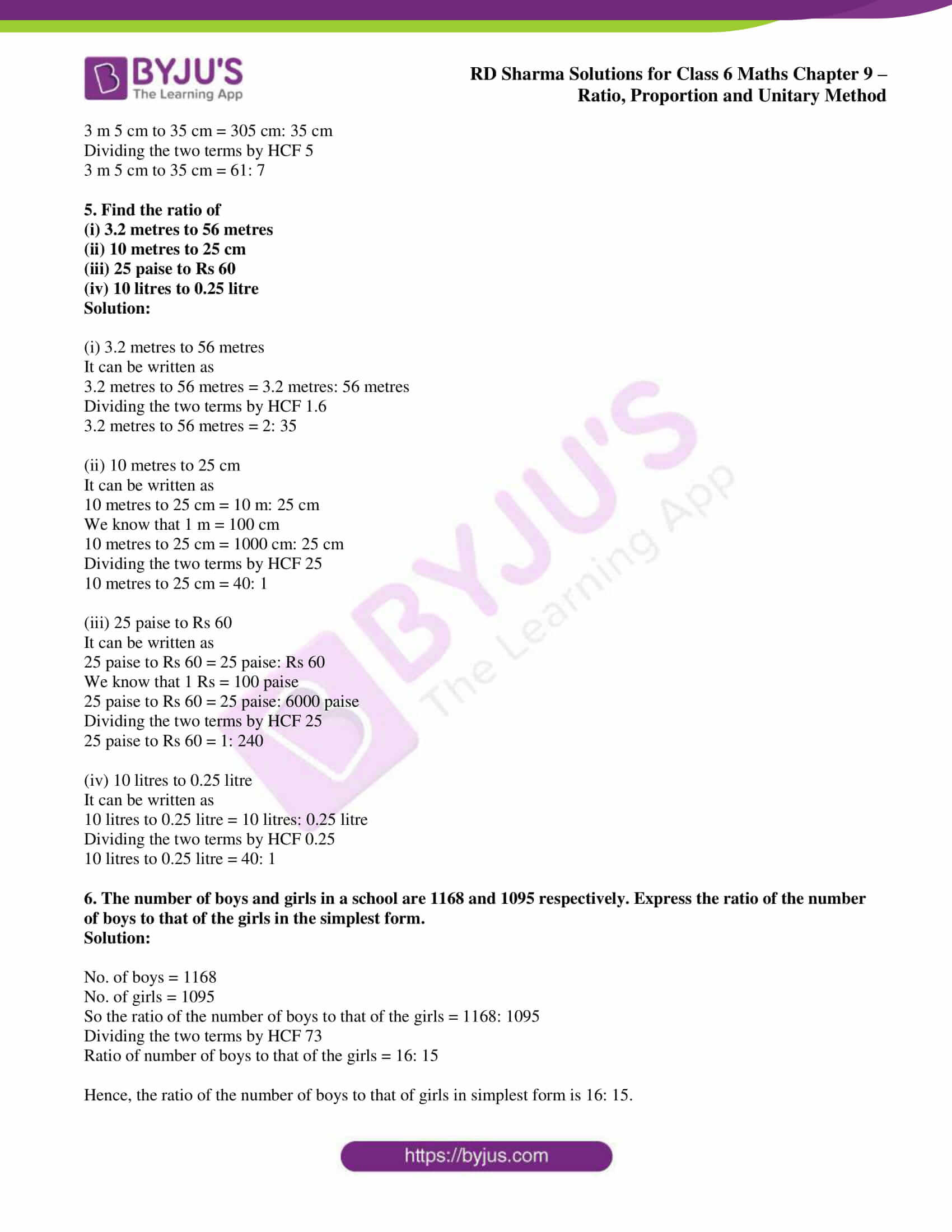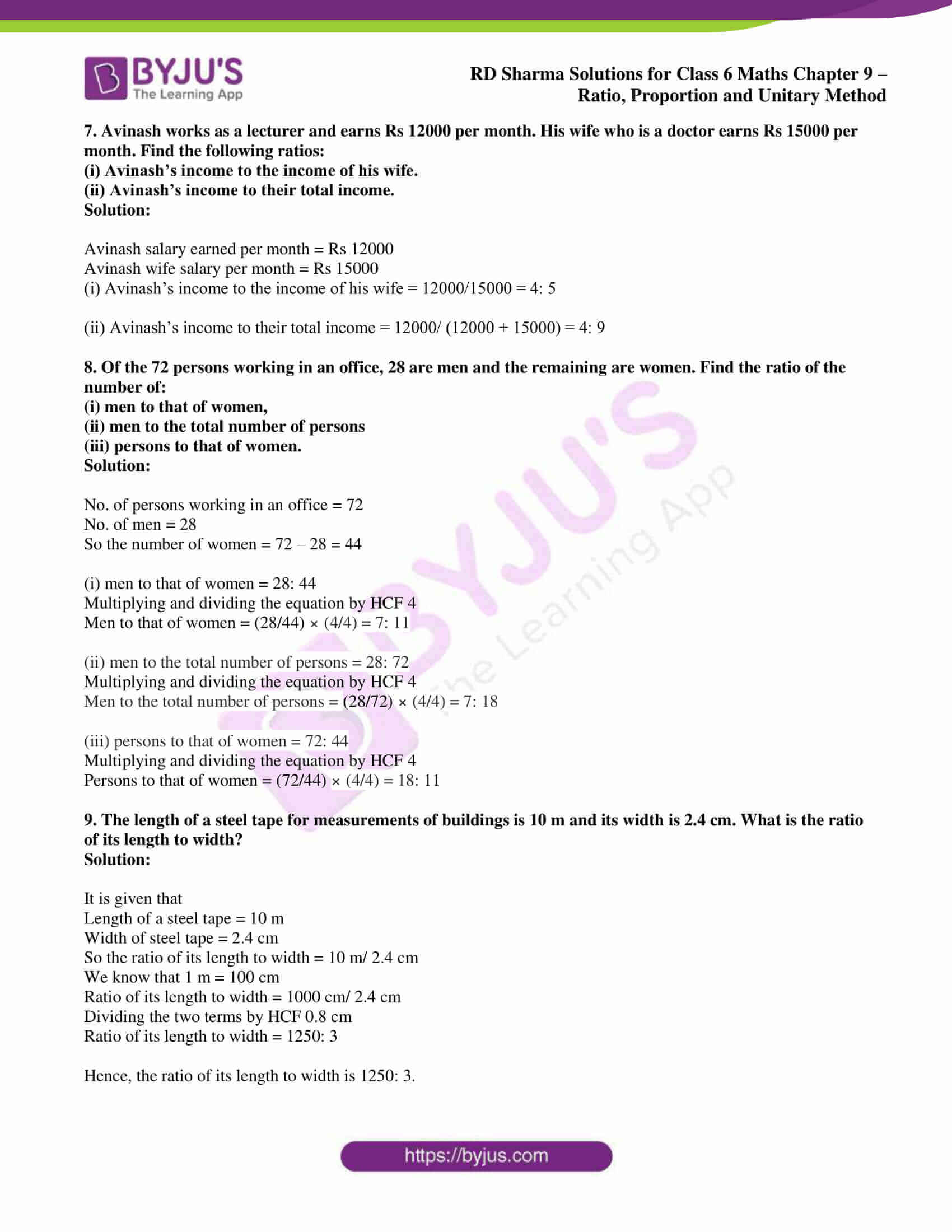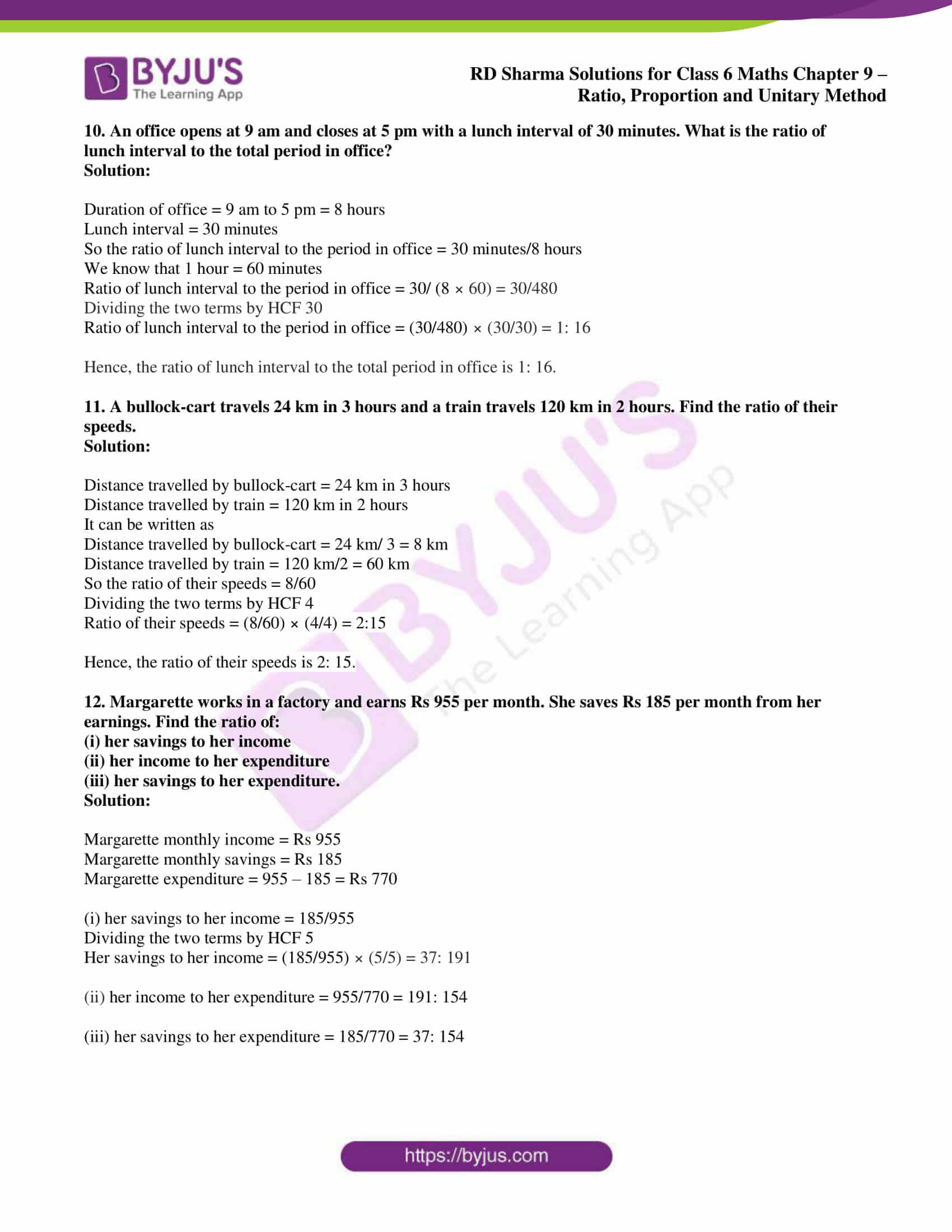### Access answers to Maths RD Sharma Solutions for Class 6 Chapter 9: Ratio, Proportion and Unitary Method Exercise 9.1

1. Express each of the following in the language of ratios:

(i) In a class, the number of girls in the merit list of the board examination is two times that of boys.

(ii) The number of students passing mathematics test is 2/3 of the number that appeared.

Solution:

(i) Ratio of the number of girls to that of boys in the merit list is 2: 1.

(ii) Ratio of the number of students passing a mathematics test to that of total students appearing in the test is 2: 3.

2. Express the following ratios in language of daily life:

(i) The ratio of the number of bad pencils to that of good pencils produced in a factory is 1: 9.

(ii) In India, the ratio of the number of villages to that of cities is about 2000: 1.

Solution:

(i) The number of bad pencils produced in a factory is 1/9 of the number of good pencils produced in the factory.

(ii) The number of villages is 2000 times that of cities in India.

3. Express each of the following ratios in its simplest form:

(i) 60: 72

(ii) 324: 144

(iii) 85: 391

(iv) 186: 403

Solution:

(i) 60: 72

It can be written as 60/72

We know that the HCF of 60 and 72 is 12

By dividing the term by 12 we get

(60/72) × (12/12) = 5/6

So we get 60: 72 = 5: 6

(ii) 324: 144

It can be written as 324/144

We know that the HCF of 324 and 144 is 36

By dividing the term by 36 we get

(324/144) × (36/36) = 9/4

So we get 324: 144 = 9: 4

(iii) 85: 391

It can be written as 85/391

We know that the HCF of 85 and 391 is 17

By dividing the term by 17 we get

(85/391) × (17/17) = 5/23

So we get 85: 391 = 5: 23

(iv) 186: 403

It can be written as 186/403

We know that the HCF of 186 and 403 is 31

By dividing the term by 31 we get

(186/403) × (31/31) = 6/13

So we get 186: 403 = 6: 13

4. Find the ratio of the following in the simplest form:

(i) 75 paise to Rs 3

(ii) 35 minutes to 45 minutes

(iii) 8 kg to 400 gm

(iv) 48 minutes to 1 hour

(v) 2 metres to 35 cm

(vi) 35 minutes to 45 seconds

(vii) 2 dozen to 3 scores

(viii) 3 weeks to 3 days

(ix) 48 min to 2 hours 40 min

(x) 3 m 5 cm to 35 cm

Solution:

(i) 75 paise to Rs 3

It can be written as

75 paise to Rs 3 = 75 paise: Rs 3

We know that 1 Rs = 100 paise

So we get

75 paise to Rs 3 = 75 paise: 300 paise

Dividing the two terms by HCF 75

75 paise to Rs 3 = 1: 4

(ii) 35 minutes to 45 minutes

It can be written as

35 minutes to 45 minutes = 35 minutes: 45 minutes

Dividing the two terms by HCF 5

35 minutes to 45 minutes = 7: 9

(iii) 8 kg to 400 gm

It can be written as

8 kg to 400 gm = 8 kg: 400 gm

We know that 1 kg = 1000 gm

So we get

8 kg to 400 gm = 8000 gm: 400 gm

Dividing the two terms by HCF 400

8 kg to 400 gm = 20: 1

(iv) 48 minutes to 1 hour

It can be written as

48 minutes to 1 hour = 48 minutes: 1 hour

We know that 1 hour = 60 minutes

So we get

48 minutes to 1 hour = 48 minutes: 60 minutes

Dividing the two terms by HCF 12

48 minutes to 1 hour = 4: 5

(v) 2 metres to 35 cm

It can be written as

2 metres to 35 cm = 2 metres: 35 cm

We know that 1 m = 100 cm

So we get

2 metres to 35 cm = 200 cm: 35 cm

Dividing the two terms by HCF 5

2 metres to 35 cm = 40: 7

(vi) 35 minutes to 45 seconds

It can be written as

35 minutes to 45 seconds = 35 minutes: 45 seconds

We know that 1 minute = 60 seconds

So we get

35 minutes to 45 seconds = 2100 seconds: 45 seconds

Dividing the two terms by HCF 15

35 minutes to 45 seconds = 140: 3

(vii) 2 dozen to 3 scores

It can be written as

2 dozen to 3 scores = 2 dozen: 3 scores

We know that 1 dozen = 12 score = 20

So we get

2 dozen to 3 scores = 24: 60

Dividing the two terms by HCF 12

2 dozen to 3 scores = 2: 5

(viii) 3 weeks to 3 days

It can be written as

3 weeks to 3 days = 3 weeks: 3 days

We know that 1 week = 7 days

So we get

3 weeks to 3 days = 21 days: 3 days

Dividing the two terms by HCF 3

3 weeks to 3 days = 7: 1

(ix) 48 min to 2 hours 40 min

It can be written as

48 min to 2 hours 40 min = 48 min: 2 hours 40 min

We know that 1 hour = 60 minutes

So we get

48 min to 2 hours 40 min = 48 min: 160 min

Dividing the two terms by HCF 16

48 min to 2 hours 40 min = 3: 10

(x) 3 m 5 cm to 35 cm

It can be written as

3 m 5 cm to 35 cm = 3 m 5 cm: 35 cm

We know that 1 m = 100 cm

So we get

3 m 5 cm to 35 cm = 305 cm: 35 cm

Dividing the two terms by HCF 5

3 m 5 cm to 35 cm = 61: 7

5. Find the ratio of

(i) 3.2 metres to 56 metres

(ii) 10 metres to 25 cm

(iii) 25 paise to Rs 60

(iv) 10 litres to 0.25 litre

Solution:

(i) 3.2 metres to 56 metres

It can be written as

3.2 metres to 56 metres = 3.2 metres: 56 metres

Dividing the two terms by HCF 1.6

3.2 metres to 56 metres = 2: 35

(ii) 10 metres to 25 cm

It can be written as

10 metres to 25 cm = 10 m: 25 cm

We know that 1 m = 100 cm

10 metres to 25 cm = 1000 cm: 25 cm

Dividing the two terms by HCF 25

10 metres to 25 cm = 40: 1

(iii) 25 paise to Rs 60

It can be written as

25 paise to Rs 60 = 25 paise: Rs 60

We know that 1 Rs = 100 paise

25 paise to Rs 60 = 25 paise: 6000 paise

Dividing the two terms by HCF 25

25 paise to Rs 60 = 1: 240

(iv) 10 litres to 0.25 litre

It can be written as

10 litres to 0.25 litre = 10 litres: 0.25 litre

Dividing the two terms by HCF 0.25

10 litres to 0.25 litre = 40: 1

6. The number of boys and girls in a school are 1168 and 1095 respectively. Express the ratio of the number of boys to that of the girls in the simplest form.

Solution:

No. of boys = 1168

No. of girls = 1095

So the ratio of the number of boys to that of the girls = 1168: 1095

Dividing the two terms by HCF 73

Ratio of number of boys to that of the girls = 16: 15

Hence, the ratio of the number of boys to that of girls in simplest form is 16: 15.

7. Avinash works as a lecturer and earns Rs 12000 per month. His wife who is a doctor earns Rs 15000 per month. Find the following ratios:

(i) Avinash’s income to the income of his wife.

(ii) Avinash’s income to their total income.

Solution:

Avinash salary earned per month = Rs 12000

Avinash wife salary per month = Rs 15000

(i) Avinash’s income to the income of his wife = 12000/15000 = 4: 5

(ii) Avinash’s income to their total income = 12000/ (12000 + 15000) = 4: 9

8. Of the 72 persons working in an office, 28 are men and the remaining are women. Find the ratio of the number of:

(i) men to that of women,

(ii) men to the total number of persons

(iii) persons to that of women.

Solution:

No. of persons working in an office = 72

No. of men = 28

So the number of women = 72 – 28 = 44

(i) men to that of women = 28: 44

Multiplying and dividing the equation by HCF 4

Men to that of women = (28/44) × (4/4) = 7: 11

(ii) men to the total number of persons = 28: 72

Multiplying and dividing the equation by HCF 4

Men to the total number of persons = (28/72) × (4/4) = 7: 18

(iii) persons to that of women = 72: 44

Multiplying and dividing the equation by HCF 4

Persons to that of women = (72/44) × (4/4) = 18: 11

9. The length of a steel tape for measurements of buildings is 10 m and its width is 2.4 cm. What is the ratio of its length to width?

Solution:

It is given that

Length of a steel tape = 10 m

Width of steel tape = 2.4 cm

So the ratio of its length to width = 10 m/ 2.4 cm

We know that 1 m = 100 cm

Ratio of its length to width = 1000 cm/ 2.4 cm

Dividing the two terms by HCF 0.8 cm

Ratio of its length to width = 1250: 3

Hence, the ratio of its length to width is 1250: 3.

10. An office opens at 9 am and closes at 5 pm with a lunch interval of 30 minutes. What is the ratio of lunch interval to the total period in office?

Solution:

Duration of office = 9 am to 5 pm = 8 hours

Lunch interval = 30 minutes

So the ratio of lunch interval to the period in office = 30 minutes/8 hours

We know that 1 hour = 60 minutes

Ratio of lunch interval to the period in office = 30/ (8 × 60) = 30/480

Dividing the two terms by HCF 30

Ratio of lunch interval to the period in office = (30/480) × (30/30) = 1: 16

Hence, the ratio of lunch interval to the total period in office is 1: 16.

11. A bullock-cart travels 24 km in 3 hours and a train travels 120 km in 2 hours. Find the ratio of their speeds.

Solution:

Distance travelled by bullock-cart = 24 km in 3 hours

Distance travelled by train = 120 km in 2 hours

It can be written as

Distance travelled by bullock-cart = 24 km/ 3 = 8 km

Distance travelled by train = 120 km/2 = 60 km

So the ratio of their speeds = 8/60

Dividing the two terms by HCF 4

Ratio of their speeds = (8/60) × (4/4) = 2:15

Hence, the ratio of their speeds is 2: 15.

12. Margarette works in a factory and earns Rs 955 per month. She saves Rs 185 per month from her earnings. Find the ratio of:

(i) her savings to her income

(ii) her income to her expenditure

(iii) her savings to her expenditure.

Solution:

Margarette monthly income = Rs 955

Margarette monthly savings = Rs 185

Margarette expenditure = 955 – 185 = Rs 770

(i) her savings to her income = 185/955

Dividing the two terms by HCF 5

Her savings to her income = (185/955) × (5/5) = 37: 191

(ii) her income to her expenditure = 955/770 = 191: 154

(iii) her savings to her expenditure = 185/770 = 37: 154